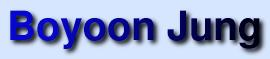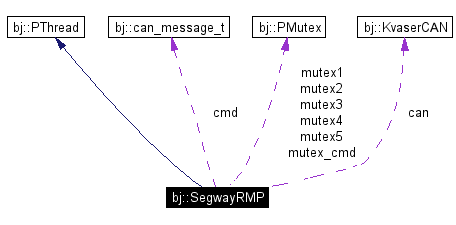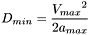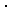Boyoon Jung's Library Manual

# bj::SegwayRMP Class Reference [Robot Programming]

A device driver for Segway RMP robots. More...

#include <segwayrmp.h>

Inherits bj::PThread.

Inheritance diagram for bj::SegwayRMP:[legend]
Collaboration diagram for bj::SegwayRMP:[legend]
List of all members.

## Public Member Functions

SegwayRMP::SegwayRMP (void)
A constructor.
SegwayRMP::~SegwayRMP (void)
A destructor.
bool setLinearVelocity (double velocity)
Set the linear velocity.
bool setAngularVelocity (double velocity)
Set the angular velocity.
bool setVelocity (double linear, double angular)
Set the linear & angular velocities.
bool stop (void)
Stop the robot.
bool resetRightOdometer (void)
Reset the right-wheel odometer.
bool resetLeftOdometer (void)
Reset the left-wheel odometer.
bool resetOdometer (void)
Reset the wheel odometers.
bool resetAngularPosition (void)
Reset the angular position.
bool resetLinearPosition (void)
Reset the linear position.
bool resetPosition (void)
Reset the positions.
bool resetAllIntegrators (void)
Reset all integrators.
bool setMaxVelocityScale (double scale)
Set the maximum velocity scale factor.
bool setMaxAccelerationScale (double scale)
Set the maximum acceleration scale factor.
bool setMaxTurnRateScale (double scale)
Set the maximum turn rate scale factor.
bool setGainSchedule (unsigned short schedule)
Set the gain schedule.
bool setCurrentLimitScale (double scale)
Set the current limit scale factor.
bool shutdown (void)
Disable power immediately to the motors.
int battery (void)
Return the latest battery gauge.
double roll (void)
Return the latest roll angle.
double pitch (void)
Return the latest pitch angle.
void angle (double &r, double &p)
Return the latest roll & pitch angles.
double rollRate (void)
Return the latest roll rate.
double pitchRate (void)
Return the latest pitch rate.
double yawRate (void)
Return the latest yaw rate.
void rate (double &r, double &p, double &y)
Return the latest roll, pitch & yaw rates.
double servoFrame (void)
Return the servo frames.
double velocityLeft (void)
Return the velocity of the left wheel.
double velocityRight (void)
Return the velocity of the right wheel.
void velocity (double &l, double &r)
Return wheel velocities.
double odometerLeft (void)
Return the odometry data of the left wheel.
double odometerRight (void)
Return the odometry data of the right wheel.
void odometer (double &l, double &r)
Return wheel odometry data.
double linearPosition (void)
Return the integrated linear position.
double angularPosition (void)
Return the integrated angular position.
void position (double &linear, double &angular)
Return the integrated position.
double x (void)
Return the X position in the odometry coordinates.
double y (void)
Return the Y position in the odometry coordinates.
double heading (void)
Return the yaw position in the odometry coordinates.

## Detailed Description

A device driver for Segway RMP robots.

SegwayRMP class provides an interface to Segway RMP robots.

## Constructor & Destructor Documentation

 bj::SegwayRMP::SegwayRMP::~SegwayRMP ( void )
 A destructor.

## Member Function Documentation

 void bj::SegwayRMP::angle ( double & r, double & p )  [inline]

Return the latest roll & pitch angles.

Parameters:
 [out] r Roll angle in degree/second. [out] p Pitch angle in degree/second.

 double bj::SegwayRMP::angularPosition ( void )  [inline]
 Return the integrated angular position. Returns:The integrated angular position in degree/second in counter-clockwise.

 int bj::SegwayRMP::battery ( void )  [inline]
 Return the latest battery gauge.

 double bj::SegwayRMP::heading ( void )  [inline]
 Return the yaw position in the odometry coordinates. Returns:The heading in radian in the odometry coordinate system.

 double bj::SegwayRMP::linearPosition ( void )  [inline]
 Return the integrated linear position. Returns:The integrated linear position in meter/second.

 void bj::SegwayRMP::odometer ( double & l, double & r )  [inline]

Return wheel odometry data.

Parameters:
 [out] l The odometry data of the left wheel in meter. [out] r The odometry data of the right wheel in meter.

 double bj::SegwayRMP::odometerLeft ( void )  [inline]
 Return the odometry data of the left wheel. Returns:The odometry data of the left wheel in meter.

 double bj::SegwayRMP::odometerRight ( void )  [inline]
 Return the odometry data of the right wheel. Returns:The odometry data of the right wheel in meter.

 double bj::SegwayRMP::pitch ( void )  [inline]
 Return the latest pitch angle. Returns:Pitch angle in degree/second.

 double bj::SegwayRMP::pitchRate ( void )  [inline]
 Return the latest pitch rate. Returns:Pitch rate in degree/second.

 void bj::SegwayRMP::position ( double & linear, double & angular )  [inline]

Return the integrated position.

Parameters:
 [out] linear The integrated linear position. [out] angular The integrated angular position in counter-clockwise.

 void bj::SegwayRMP::rate ( double & r, double & p, double & y )  [inline]

Return the latest roll, pitch & yaw rates.

Parameters:
 [out] r Roll rate in degree/second. [out] p Pitch rate in degree/second. [out] y Yaw rate in degree/second.

 bool bj::SegwayRMP::resetAllIntegrators ( void )
 Reset all integrators.

 bool bj::SegwayRMP::resetAngularPosition ( void )
 Reset the angular position.

 bool bj::SegwayRMP::resetLeftOdometer ( void )
 Reset the left-wheel odometer.

 bool bj::SegwayRMP::resetLinearPosition ( void )
 Reset the linear position.

 bool bj::SegwayRMP::resetOdometer ( void )
 Reset the wheel odometers.

 bool bj::SegwayRMP::resetPosition ( void )
 Reset the positions.

 bool bj::SegwayRMP::resetRightOdometer ( void )
 Reset the right-wheel odometer.

 double bj::SegwayRMP::roll ( void )  [inline]
 Return the latest roll angle. Returns:Roll angle in degree/second.

 double bj::SegwayRMP::rollRate ( void )  [inline]
 Return the latest roll rate. Returns:Roll rate in degree/second.

 bj::SegwayRMP::SegwayRMP::SegwayRMP ( void )
 A constructor.

 double bj::SegwayRMP::servoFrame ( void )  [inline]
 Return the servo frames.

 bool bj::SegwayRMP::setAngularVelocity ( double velocity )

Set the angular velocity.

Parameters:
 velocity Angular velocity in degree/second.

 bool bj::SegwayRMP::setCurrentLimitScale ( double scale )

Set the current limit scale factor.

The available wheel torque may be limited using a normalization factor (Full torque=256, No torque=0). The peak torque of the each wheel is 122 Newton-meters. Dynamic balancing rquires transient torque to accelerate, decelerate and traverse small obstacles. However, the amount of torque required depends on the environment, task and payload and reducing available torque may be prudent in some cases. In balance mode, reducing the torque too low will result in the RMP falling over during a transient.

Parameters:
 scale A scale factor [0.0 ~ 1.0].

 bool bj::SegwayRMP::setGainSchedule ( unsigned short schedule )

Set the gain schedule.

There are three controller gain schedules, each optimized for a different payload configuration.

• Controller Gain Schedule 0 ("Light") : This controller has been tuned for a 50 lb payload located at the top plate. This configuration may be created by using (2) of the 25 lb ballast plates. As payload is added, the ballast plates should be removed.
• Controller Gain Schedule 1 ("Tall") : This controller has been tuned for a 25 lb payload located at the top plate and a 25 lb payload located 75 cm above the top plate.
• Controller Gain Schedule 2 ("Heavy") : This controller has been tuned for a 100 lb payload located at the top plate.

Parameters:
 schedule A controller gain schedule [0, 1, or 2].

 bool bj::SegwayRMP::setLinearVelocity ( double velocity )

Set the linear velocity.

Parameters:
 velocity Linear velocity in meter/second.

 bool bj::SegwayRMP::setMaxAccelerationScale ( double scale )

Set the maximum acceleration scale factor.

The minimum stopping distance may be calculated from the maximum velocity,, and maximum acceleration,:Thus, large velocities with small accelerations produce large stoppping distances. Choose your acceleration limits with care, considering both the need to stop quickly and the top speed you have chosen.

Parameters:
 scale A scale factor [0.0 ~ 1.0].

 bool bj::SegwayRMP::setMaxTurnRateScale ( double scale )

Set the maximum turn rate scale factor.

A turning scale factor parameter may be used to limit the maximum lateral acceleration of the RMP. This may be particularly useful with tall payloads, to prevent the RMP from tipping. In most cases though, higher turning sensitivity is useful for navigating around obstacles.

Parameters:
 scale A scale factor [0.0 ~ 1.0].

 bool bj::SegwayRMP::setMaxVelocityScale ( double scale )

Set the maximum velocity scale factor.

The minimum stopping distance may be calculated from the maximum velocity,, and maximum acceleration,:Thus, large velocities with small accelerations produce large stoppping distances. Choose your acceleration limits with care, considering both the need to stop quickly and the top speed you have chosen.

Parameters:
 scale A scale factor [0.0 ~ 1.0].

 bool bj::SegwayRMP::setVelocity ( double linear, double angular )

Set the linear & angular velocities.

Parameters:
 linear Linear velocity in meter/second. angular Angular velocity in degree/second.

 bool bj::SegwayRMP::shutdown ( void )
 Disable power immediately to the motors. Use of this command will cause the balancing function of the Segway RMP to stop. Use this message with caution.

 bool bj::SegwayRMP::stop ( void )  [inline]
 Stop the robot.

 void bj::SegwayRMP::velocity ( double & l, double & r )  [inline]

Return wheel velocities.

Parameters:
 [out] l The velocity of the left wheel in meter/second. [out] r The velocity of the right wheel in meter/second.

 double bj::SegwayRMP::velocityLeft ( void )  [inline]
 Return the velocity of the left wheel. Returns:The velocity of the left wheel in meter/second.

 double bj::SegwayRMP::velocityRight ( void )  [inline]
 Return the velocity of the right wheel. Returns:The velocity of the right wheel in meter/second.

 double bj::SegwayRMP::x ( void )  [inline]
 Return the X position in the odometry coordinates. Returns:The X position in meter in the odometry coordinate system.

 double bj::SegwayRMP::y ( void )  [inline]
 Return the Y position in the odometry coordinates. Returns:The Y position in meter in the odometry coordinate system.

 double bj::SegwayRMP::yawRate ( void )  [inline]
 Return the latest yaw rate. Returns:Yaw rate in degree/second.

The documentation for this class was generated from the following file:
ResearchProjectsPublications

ToolsBjLib ManualPlayer/StagePyPlayerMicroStrain IMUGPS/DGPSParticle Filter

PersonalWelcomeCurriculum VitaeResumeContact

Outside LinksUSCUSC CS DeptCRESRobotics LabRESL